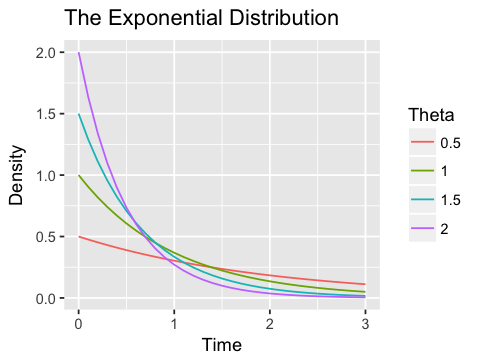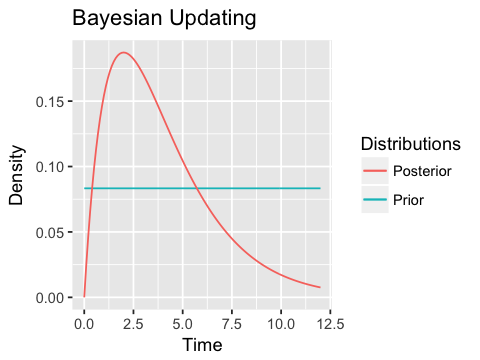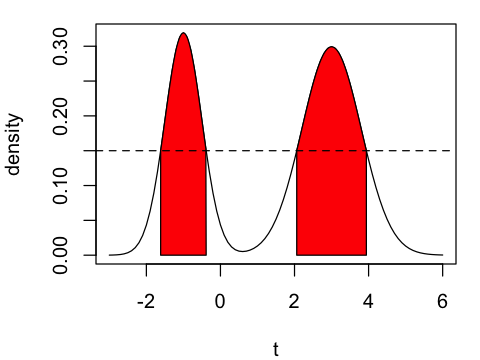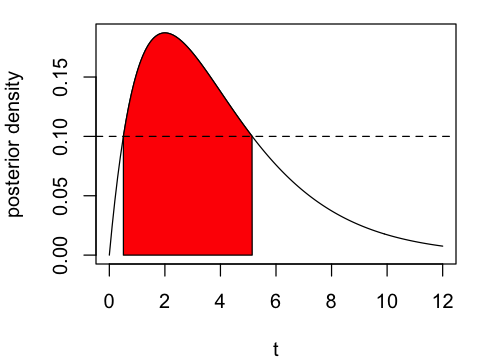Sept. 5, 2018 $\newcommand{\bs}{\boldsymbol}$ $\newcommand{\argmin}{\underset{\bs{#1}}{\text{arg min}}\,}$ $\newcommand{\argmax}{\underset{\bs{#1}}{\text{arg max}}\,}$ $\newcommand{\tr}{^{\top}}$ $\newcommand{\norm}{\left|\left|\,#1\,\right|\right|}$ $\newcommand{\given}{\,|\,}$

Statisticians are well trained in the frequentist philosophy, which assumes that the population parameter is a fixed constant, and the chance experiment associated with that parameter can be repeated many times. The Bayesian approach goes the other way. The population parameter is viewed as a random variable, whose distribution represents our uncertainty about the unknown parameter. In this post I would like to discuss the fundamental difference between these two approaches. Let's first start with a motivating example.

Example Yesterday, I spent 30 min at Sikes' Hall waiting for the bus to arrive. What is a reasonable probability model for $\theta$, the expected number of busses that arrives at the bus stop per hour?

Let $T$ denote the waiting time until the bus arrives. It is reasonable to assume that $T$ follows an exponential distribution with rate parameter $\theta$. So the probability density function is

$$p_{\text{model}}(t; \theta) = \theta e^{-\theta t}\, I(x\geq0) \tag{1}$$

Let's plot the density of the exponential distribution for various values of $\theta$.

I really love R's base plot for its extremely simple syntax. With a few lines of code we can quickly explore a problem without worrying too much about unnecessary details.

In :
options(repr.plot.width=6, repr.plot.height=4)

par(mfrow=c(2,2), mar = c(4, 4, 1, 1))
t <- seq(0, 3, by=0.1)
theta <- c(0.5, 1, 1.5, 2)

for (i in 1:4) {
plot(t, dexp(t, theta[i]), t='l', ylab='density', ylim=c(0, 2))
}Alternatively, we can use ggplot2 to produce a more fancy plot.

In :
library(dplyr)
library(data.table)
library(ggplot2)
library(reshape2) # for the melt() function

In :
options(repr.plot.width=4, repr.plot.height=3)

# Creating four different arrays of values
t <- seq(0, 3, by=0.1)
df.1 <- dexp(t, 0.5)
df.2 <- dexp(t, 1)
df.3 <- dexp(t, 1.5)
df.4 <- dexp(t, 2)

# Combine them into a single data table
data <- cbind(t, df.1, df.2, df.3, df.4) %>% as.data.table()
names(data) <- c('t', '0.5', '1', '1.5', '2')

# The melt function is nice for plotting as it converts a data frame into a
# single array with two additional columns "value" and "variable"
data_long <- melt(data, id="t")
ggplot(data_long, aes(x=t, y=value, color=variable)) +
geom_line() +
labs(title = "The Exponential Distribution", x = "Time", y = "Density") +
guides(color=guide_legend(title="Theta"))From these plots we see that as the value of $\theta$ increases, the density gets higher around 0. The $\theta$ is also referred to as the arrival intensity of a Poisson process.

## Frequentist Approach¶

From my earlier post, the most popular frequentist apporach is by minimizing the cross entropy between the empirical distribution and the modeled distribution defined in (1). In this case, the empirical distribution is just $\widehat{p}(0.5)=1$. Hence, we want to minimize

\begin{aligned} E_{t\sim \widehat{p}} -\log p_{\text{model}}(t; \theta) &= -\log p_{\text{model}}(0.5; \theta) \\ &= -\log\theta + 0.5\theta \end{aligned}

Differentiating the cross entropy and setting it equal to 0 we have the maximum likelihood extimator:

$$\widehat{\theta}_{\text{MLE}} = 2$$

In general, if we have data $t_1, t_2,..., t_n$, we can use the same procedure to derive the maximum likelihood estimator to be

$$\widehat{\theta}_{\text{MLE}} = \frac{n}{\sum_{i=1}^n t_i}.\tag{2}$$

Here we know that $\widehat{\theta}_{\text{MLE}}$ follows a scaled inverse-gamma distribution; hence we can also find an exact interval estimate using either calculus or simulation. However, it is not always possible to know the exact underlying distribution of the maximum likelihood estimator.

One of the most powerful weapon in a frequentist's arsenal is the Central Limit Theorem (CLT), which states that the sum of many independent random variables approximately follows a normal distribution. We state it more formally below.

Central Limit Theorem Let $X_1, X_2,...$ be a sequence of iid random variables with finite first and second moments, then

$$\sqrt{n}\,(\bar{X}_n - \mu) \overset{d}{\to} N(0, \sigma^2).$$

A generalization of the CLT is called the Delta Method, which is given below.

Delta Method Let $Y_n$ be a sequence of random variables that satisfies $\sqrt{n} (Y_n-\theta) \overset{d}{\to} N(0, \sigma^2)$. If $g'(\theta)\neq 0$, then

$$\sqrt{n}\left[g(Y_n)-g(\theta)\right] \overset{d}{\to} N\left(0, \sigma^2[g'(\theta)]^2\right).$$

Let's apply these two theorems to (2). First note that the MLE is nothing more than the reciprocal of a sample mean. Also since each $T_i$ is exponentially distributed with both mean and standard deviation equal to $1/\theta$, the Central Limit Theorem gives

$$\sqrt{n}\,\left(\frac{1}{n}\sum_{i=1}^n t_i - \frac{1}{\theta}\right) \overset{d}{\to} N\left(0, \frac{1}{\theta^2}\right).$$

To use the Delta Method, let $g(y) = 1/y$, so that $g'(y) = -1/y^2$. So we have

$$\sqrt{n} \left( \widehat{\theta}_{\text{MLE}} - \theta \right) \overset{d}{\to} N\left(0, \frac{1}{\theta^6}\right).$$

This means as $n$ gets "large", we have that

$$\widehat{\theta}_{\text{MLE}} \overset{\text{approx}}{\sim} N\left(\theta, \frac{1}{n\theta^6} \right).$$

Using the asymptotic approximation, we can find the frequentist's greatest weapon, the $100(1-\alpha)\%$ confidence interval, which is given by

$$\widehat{\theta}_{\text{MLE}} \pm z_{1-\alpha/2} \frac{1}{\sqrt{n}\, \left(\widehat{\theta}_{\text{MLE}}\right)^3}$$

where $z_{1-\alpha/2}$ is the critical value corresponding to the $100(1-\alpha/2)$th percentile of the standard normal density.

Since $n=1$ in our example, we cannot use the CLT. Instead, we can use simulation by rewriting the estimator as

$$\widehat{\theta} = \frac{1}{T}, \quad \text{ where } T \sim \text{Exponential}(2).$$

In R we can easily simulate many such random variables.

In :
# create 100000 randomly generated estimators
set.seed(1)
1/rexp(100000, 2) -> sample

In :
# a function that returns the percentage of sample points in an interval around a point estimate
sample.percentage <- function(sample, theta=2, h=0.1) {
theta.lower <- theta - h
theta.upper <- theta + h
percentage <- mean(sample > theta.lower & sample < theta.upper)
return(percentage)
}

In :
# recursively find the confidence interval based on alpha
sample.CI <- function(sample, theta=2, h=0.1, alpha=0.05, tolerance = 0.001) {
percentage <- sample.percentage(sample, theta, h)
if (percentage < 1 - alpha - tolerance) {
h <- h + 0.1
sample.CI(sample, h=h)
} else if (percentage > 1 - alpha + tolerance) {
h <- h - 0.1
sample.CI(sample, h=h)
} else {
return(c(max(0, theta-h), theta+h, percentage))
}
}

In :
print(sample.CI(sample))

  0.00000 38.60000  0.94903


So based on only one data point, the 95% confidence interval is found to be apprximately $[0, 38]$. The biggest downside of the frequentist confidence interval is that it is difficult to interpret. One is tempted to think that there is a 95% probability that the true value of $\theta$ lies in the interval $[0, 38]$. This is incorrect since the frequentist paradigm deems $\theta$ to be a fixed parameter, so it is meaningless to mention such probability. Instead, we interpret it this way:

If we repeat the chance experiment many times (hence the name "frequentist"), we are expected to see that about 95% of the confidence intervals will cover the true value $\theta$.

## Bayesian Approach¶

Denote by $\bs{t}$ the data that we observed, the Bayesian approach relies on the Bayes' Theorem:

\begin{aligned} p(\theta \given \bs{t}) &= \frac{p(\theta)p(\bs{t}\given \theta)}{p(\bs{t})} \\ &\propto p(\theta)p(\bs{t}\given \theta) \end{aligned}

Here $p(\theta)$ is called the prior distribution. It represents our belief about $\theta$ prior to seeing the data $\bs{t}$. The conditional distribution $p(\theta \given \bs{t})$ is called the posterior distribution. It represents our updated belief about $\theta$ after observing the data $\bs{t}$.

When the data is limited, Bayesian statistics shines only when the prior distribution is appropriately chosen. Based on my real world experience, I know that the busses travel to and fro between two locations, which are distanced about 1 hour apart. On any given day, the maximum number of buses running simultaneously is 6. Below is a screenshot of the live map of the busses. At the time of writing, there are four busses running simultaneously.source: http://www.catbus.com/

Let's consider the best case scenario:

1. You see ALL 6 busses passing by a bus stop.
2. You wait at the bus stop for at most one hour.
3. You see 6 busses returning.
4. Since it takes one hour for the bus to travel from one end of the route to the other, you conclude that the maximum number of busses one can observe in a single hour is 12.

Hence, to be as uninformative as possitle, it is best to use a uniform prior distribution given by

$$p(\theta) = \frac{1}{12} I(\theta \in(0, 12)).$$

Under the uniform prior, the posterior density of $\theta$ is given by

$$p(\theta \given t=0.5) \propto \theta e^{-\theta/2} I(\theta \in (0, 12)).$$

To find the exact distribution, we integrate the above function:

$$\int_0^{12} \theta e^{-\theta/2} \, dx = 4-28e^{-6} \approx 3.9306$$

Hence the normalizing constant should be approximately $1/3.9306 \approx 0.2544$, and the posterior density is given by

$$p(\theta \given t=0.5) \approx 0.2544 \theta e^{-\theta/2} I(\theta \in (0, 12)).$$

In :
t <- seq(0, 12, by=0.1)
prior <- rep(1/12, 121)
post <- 0.2544*t*exp(-t/2)

data <- cbind(t, prior, post) %>% as.data.table()
names(data) <- c('t', 'prior', 'posterior')

ggplot(data) +
geom_line(aes(x=t, y=prior, color="Prior"))+
geom_line(aes(x=t, y=posterior, color="Posterior")) +
labs(title = "Bayesian Updating", x = "Time", y = "Density")+
guides(color=guide_legend(title="Distributions"))Knowing the posterior distribution, we can find a "point" estimator by using either mean or median of the distribution. Here it is relatively easy to compute the mean:

$$\widehat{\theta} = \int_0^{12} 0.2544\theta^2 e^{-\theta/2}\, d\theta \approx 3.81812$$

Altenatively, we can find the median by solving

$$\int_0^{\theta} 0.2544 x e^{-x/2} dx = 0.5.$$

In R this can be accomplished by using the uniroot function.

In :
# define the integrand
f <- function(x){0.2544*x*exp(-x/2)}

# define the integral minus 0.5
integral <- function(theta){integrate(f, 0, theta)$value - 0.5} # solve for the median uniroot(f=integral, lower=0, upper=12)$root

3.30179279758604

Hence, we find that the median point estimator is

$$\widehat{\theta} \approx 3.3018.$$

We prefer the median here since the distribution is skewed, which slightly distorts the mean. In both estimates, the point estimates are higher than the frequentist estimate of 2.

What about the confidence interval? Prepare for a definition.

Definition The Bayesian credible interval, $[l(\bs{t}), u(\bs{t})]$, based on the observed data $\bs{T}=\bs{t}$ is an interval that has 95% coverage of $\theta$, i.e.,

$$P\left(l(\bs{t}) < \theta < u(\bs{t}) \given \bs{T}=\bs{t}\right) = 0.95.$$

The easiest approach to constructing a $100(1-\alpha)\%$ credible intervals is by using the equal-tailed method. The steps are summarized below.

1. First let $\alpha^* = \alpha/2$.
2. Find the values $\theta^*$ and $\theta^{**}$ such that \begin{aligned} P(\theta < \theta^*) &= \alpha^* \\ P(\theta > \theta^{**}) &= \alpha^* \end{aligned}
3. The resulting interval $[\theta^*, \theta^{**}]$ is the equal-tailed Bayesian credible interval.

To find the equal-tailed credible interval for our problem, we solve

\begin{aligned} \int_0^{\theta^*} 0.2544 x e^{-x/2} dx &= 0.025 \\ \int_{\theta^{**}}^{12} 0.2544 x e^{-x/2} dx &= 0.025. \end{aligned}

Using R this can be easily accomplished as follows.

In :
# define the integrand
f <- function(x){0.2544*x*exp(-x/2)}

# define the integral minus 0.5
integral.lower <- function(theta){integrate(f, 0, theta)$value - 0.025} integral.upper <- function(theta){integrate(f, theta, 12)$value - 0.025}

# solve for the median
ci.lower <- uniroot(f=integral.lower, lower=0, upper=12)$root ci.upper <- uniroot(f=integral.upper, lower=0, upper=12)$root

print(paste0("The 95% equal-tailed credible interval is ", "(", ci.lower, ", ",  ci.upper, ")."))

 "The 95% equal-tailed credible interval is (0.47988167307245, 9.91301438773853)."


But is this the best interval we can construct? By the frequentist preference, we want the credible interval to be as narrow as possible. This can be accomplished using the highest posterior density (HPD) region.

Definition A $100(1-\alpha)\%$ HPD region consists a subset of the support of $\theta$, denoted by $s(\bs{t})$, such that

1. $P(\theta \in s(\bs{t}) \given \bs{T}=\bs{t}) = 1-\alpha$
2. If $\theta^* \in s(\bs{t})$ and $\theta^{**} \not\in s(\bs{t})$, then $p(\theta^* \given \bs{t}) > p(\theta^{**} \given \bs{t})$.

To illustrate the concept of HPD, we generate a bimodal mixture Guassian distribution:

$$0.4\times N(-1, .5^2) + 0.6\times N(3, 0.8^2)$$

In :
par(mar = c(4, 4, 1, 1))

t <- seq(-3, 6, by=0.1)

# mixed Guassian density
dist <- function(t){0.4*dnorm(t, -1, 0.5) + 0.6*dnorm(t, 3, 0.8)}

# the mixed Guassian minus a constant (for root finding)
dist2 <- function(t){0.4*dnorm(t, -1, 0.5) + 0.6*dnorm(t, 3, 0.8) - 0.15}

# finding the intercept between y=0.15 and the mixed density
x1 <- uniroot(f=dist2, lower=-3, upper=-1)$root x2 <- uniroot(f=dist2, lower=-1, upper=1)$root
x3 <- uniroot(f=dist2, lower=1, upper=3)$root x4 <- uniroot(f=dist2, lower=3, upper=5)$root

# creating a plot with shaded region
left.x <- c(x1, seq(x1, x2, 0.01), x2)
left.y <- c(0, dist(seq(x1, x2, 0.01)), 0)

right.x <- c(x3, seq(x3, x4, 0.01), x4)
right.y <- c(0, dist(seq(x3, x4, 0.01)), 0)

plot(t, dist(t), t='l', ylab='density')
polygon(left.x, left.y, col="red")
polygon(right.x, right.y, col="red")
abline(h=0.15, lty=2)From the plot above, the $x$-values for the sharded region, whose area is $100(1-\alpha)\%$, corresponds to the HPD intervals. It can be proven that the HPD interval is the shortest amongst all $100(1-\alpha)\%$ credible intervals. For our original problem, we can do a similar plot as shown below.

In :
par(mar = c(4, 4, 1, 1))

t <- seq(0, 12, by=0.1)

# the posterior distribution
dist <- function(x){0.2544*x*exp(-x/2)}

# shifted posterior distribution for root finding
dist2 <- function(x){0.2544*x*exp(-x/2)-0.1}

# finding the intersections between y=0.1 and the posterior density
x1 <- uniroot(f=dist2, lower=0, upper=2)$root x2 <- uniroot(f=dist2, lower=2, upper=12)$root

# creating a plot with shaded region
left.x <- c(x1, seq(x1, x2, 0.01), x2)
left.y <- c(0, dist(seq(x1, x2, 0.01)), 0)

plot(t, dist(t), t='l', ylab='posterior density')
polygon(left.x, left.y, col="red")

abline(h=0.1, lty=2)The algorithm of finding the HPD interval for an unimodal distribution is easy to design.

Algorithm (Finding HPD interval)

• Find the mode $\tilde{\theta}$ of the posterior density.
• Construct a relative density by dividing the posterior density by its maximum value:

$$r(\theta) := \frac{p(\theta \given t)}{p(\tilde{\theta} \given t)}.$$

• Choose a height $h\in (0, 1)$. Find the values $a < \tilde{\theta}$ such that $r(a) = h$. Find the value $b > \tilde{\theta}$ such that $r(b) = h$.
• Calculate $P(a<\theta < b \given T=t)$.
• Adjust the value of $h$ until the probability converges to 0.95.

To implement this altorithm in R, we first create a function that returns the HPD interval and the associated probability with a fixed value of $h \in (0, 1)$.

In :
# function that calculates the HPD interval given a value of h
HPD.unimodal.h <- function(density, h=0.5, left=0, right=12) {

# finding the mode using the optimization function
argmax <- optimize(density, interval=c(left, right), maximum=TRUE)$maximum mode <- density(argmax) # creating normalized distributions r <- function(x){density(x)/mode} r2 <- function(x){r(x) - h} # finding the interval a <- uniroot(f = r2, lower=left, upper=argmax)$root
b <- uniroot(f = r2, lower=argmax, upper=right)$root # calculating the coverage probability coverage <- integrate(density, a, b)$value

return(c(a, b, coverage))
}

In :
# recursively finding the HPD interval based on alpha
HPD.unimodal <- function(density, h=0.5, left=0, right=12, alpha=0.05, tolerance = 0.001) {

# calculate coverage interval and probability given h
coverage.list <- HPD.unimodal.h(density, h, left, right)
a <- coverage.list
b <- coverage.list
coverage <- coverage.list

# adjusting the value of h to reach the acceptable tolerance level
if (coverage < 1 - alpha - tolerance) {
h <- h - 0.005
HPD.unimodal(density, h=h)
} else if (coverage > 1 - alpha + tolerance) {
h <- h + 0.005
HPD.unimodal(density, h=h)
} else {
return(coverage.list)
}
}

In :
HPD.unimodal(function(x){0.2544*x*exp(-x/2)})

1. 0.11287920736047
2. 8.83266151166199
3. 0.949466567586745

So the Bayesian HPD credible interval is found to be $[0.11, 8.83]$. Comparing that with the equal-tailed credible interval of $[0.48, 9.91]$, we see that the HPD interval has a shorter width. Finally, I want to mention that the Bayesian credible interval is doing much better than the frequentist confidence interval of $[0, 38]$ because we have added in the Bayesian model a well chosen prior distribution.